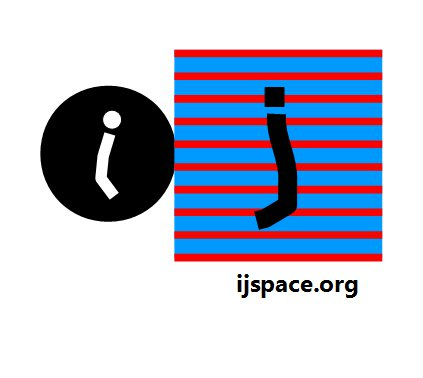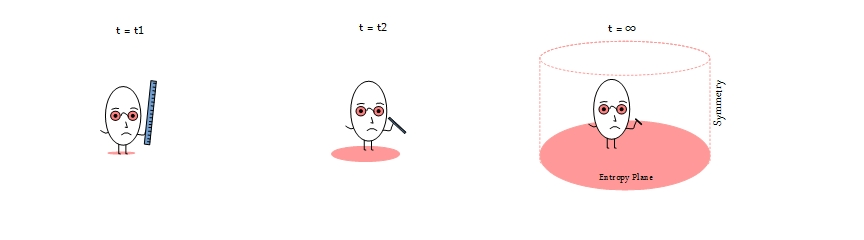# Home

 An Exact Source 18th February 2017 “Seeing there is nothing that is so troublesome to mathematical practice, nor that doth more molest and hinder calculators, than the multiplications, divisions, square and cubical extractions of great numbers... I began therefore to consider in my mind by what certain and ready art I might remove those hindrances." - John Napier, Mirifici logarithmorum canonis descriptio (1614) Reference: e:The Story of a Number by Eli Maor     "Topology is a qualitative subject where quantity is banned." - Henry Poincarè Quoted in Introduction to Graph Theory by Richard J. Trudeau. "From the point of view of logic, my report on « Exclusion principle and quantum mechanics » has no conclusion. I believe that it will only be possible to write the conclusion if a theory will be established which will determine the value of the fine-structure constant and will thus explain the atomistic structure of electricity, which is such an essential quality of all atomic sources of electric fields actually occurring in Nature." - Wolfgang Pauli, "Exclusion Principle and Quantum Mechanics" Nobel Lecture 13th December 1946. "He believed in the primacy of doubt, not as blemish on our ability to know but as the essence of knowing." - James Gleick on Feynman Quoted in Feynman lectures on computation edited by Hey and Allen. Defining a source is a subtle business.  Anything or any object can be a source.  For example sun is a source of energy, but then so is an electron or a collection of electrons i.e battery.  In real life a bank can be a source of finance.  There is no specific definition of source except probably for a consensus that it just exists.  A good source is one which lasts longer.  Well then, what is an "exact" source?  Can it be a source which exists for an infinite time?  Is the existence ad infinitum a good enough criterion?  We will define the criteria for an exact-source in a discrete measurement space we call j-space, then we will look into the measurement of a source.  (For the rest of the article, we will use the word source to represent either an infinite-source or an exact-source.)     We restrict our interpretation of a source within the context of the information space, however we also stay ambiguous by not saying what this information means.  Next we place a condition that for a source to exist an "observer" must also exist, who will measure the source to best of its capacity defined by the parameter v/c.  What we have just said, is that an "interaction" must also exist for a source to exist, since the observer exists and because without an interaction a measurement can not take place.     An example is sun, where the macroscopic observer ObsM is able to measure information such as its physical parameters, its composition, its location in galaxy etc.  The observer can also predict to within a reasonable approximation what would happen to sun in future.  Clearly sun can not be a source simply because it has a finite life-time.  However for the argument sake let us assume that the sun would have existed for an infinite time in our measurement metric.  Can it then be considered an source?  Possibly, but infinite measurements on the time-axis, is not a satisfactory enough criterion for defining a source.  For the existence of a source, the following conditions are satisfied:     i) For a source to exist an observer must exist.  Since the observer must make measurements hence an interaction must also exist, as a measurement can not be performed without an interaction.     ii) The observer defining the measurement metric for the measurement of the source, is the maximum capacity observer Obsc (v/c ~ 1).    iii) More importantly, the capacity of the observer is not constant.  Instead the observer's capacity to measure the source, is continuously decreasing with the progression of time, in the observer's frame of reference.  The continuously decreasing capacity (v/c) does not mean that v is decreasing and c is constant.  It means that the capacity of the measurement metric is continuously decreasing while measuring a source.  The v/c ratio remains intact, it is the information about the source, contained in each consecutive measurement, which is continuously decreasing.1By doing this we have characterized the only entity we know of, that is the "observer" itself.  We do not need to know the nature of the infinite-source, as long as we know the nature of the interaction and results obtained from the interaction.  So the question now becomes what is this interaction telling us about the predicament we are in?     To answer this question we must revert back to a fundamental problem posed by the great Pauli himself, that is to explain the significance of the fine-structure constant, characterizing the electron-photon coupling.  We summarize previously reported results in the context of the present discussion as follows: a) The information measured at an infinitesimally-later instant (t = 0+), is related to information contained within the initial state (t = 0) as,   I(t = 0+)    ∝    I(t = 0) × e-q The exponential function is selected, because its derivative is equal to itself and its initial value for q = 0, is 1 (or δ-function).  The variable q is allowed to take only the positive integer values.  Therefore the information structures being measured correspond to q = 1, 2 , 3 ,....etc.  These information structures are proportional to e-1, e-2, e-3 and so on.  This is how the concept of source is introduced mathematically.  b) If I(t = 0) is a δ-function then I(t = 0+) is likely to be a stable probability distribution.2  In other words, in j-space the progression of measurements with respect to time is, from δ-function ( ≡ complete information in one measurement) to a probability distribution ( ≡ complete information if and only if the measurements can be made up to the instant t = ∞). c) We note that both I(t = 0) and I(t = 0+) are measured values.  Does it mean that I(t = 0) represents the source?  No, all we are saying is that I(t = 0) is the information content an observer could measure at the instant t = 0.  The observer does not have capacity to measure even δi with complete precision, otherwise the entropy would not have kicked in for the observer leading to a deteriorating measurement metric in j-space to begin with.  The source will remain indeterminate.  The relationship between the source, i-space, j-space and corresponding observers is shown in the following diagram:The i-space contains only an infinitesimal amount of information from the source, which then is equivalent to an infinite information space for the observer ObsM.     The definition of the source in context to an observer, must be independent of different theories from different observers, used to describe the entrails of Humpty Dumpty also known as universe.  It is difficult to imagine that the universe, the perceived description of the physical reality for us, is a bloating carcass, and that the explanation of the universe based on our severely limited organic capacities, will provide an exact description of the source.    Is it possible to visualize a source as a point or the origin?  If that is the case, then how are the stable structures formed?  To understand the formation of the stable structures we need a scheme for optimization, a scheme which is independent of the physical constants.3  We can worry about the book-keeping of the resources within the measurement metric for q = 3 state, later. _______________________ 1. Note that we have just introduced a logarithmic relationship, although we did not know the base value.  The base value of 'e' is introduced in the section-(a). 2. A brief summary of stable distributions in j-space, is provided in the footnotes here. 3. Geometry or Topology only. Previous Blogs:   Nutshell-2016 Quanta-II Quanta-I EPR Paradox-II   EPR Paradox-I    Nutshell-2015